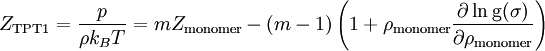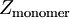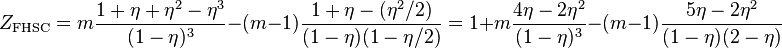# Wertheim's first order thermodynamic perturbation theory (TPT1)$Z_{\rm TPT1} = \frac{p}{\rho k_BT}= mZ_{\rm monomer}- (m-1)\left( 1 + \rho_{\rm monomer}\frac{\partial \ln {\rm g}(\sigma)}{\partial \rho_{\rm monomer}}\right)$
where$Z_{\rm monomer}$ is the equation of state of the monomer system and m is the number of monomers in the chains.
For example, in the study of the flexible hard sphere chain model one can use the Carnahan-Starling equation of state for$Z_{\rm monomer}$, leading to$Z_{\rm FHSC} = m \frac{ 1 + \eta + \eta^2 - \eta^3 }{(1-\eta)^3 } - (m-1) \frac{1+\eta-( \eta^2/2)}{(1-\eta)(1-\eta/2)}= 1+ m \frac{ 4\eta -2 \eta^2 }{(1-\eta)^3 } - (m-1) \frac{5\eta-2 \eta^2}{(1-\eta)(2-\eta)}$